x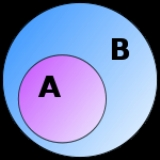Event (probability theory)Encyclopedia
In probability theory
Probability theory
Probability theory is the branch of mathematics concerned with analysis of random phenomena. The central objects of probability theory are random variables, stochastic processes, and events: mathematical abstractions of non-deterministic events or measured quantities that may either be single...

, an event is a set of outcomes (a subset
Subset
In mathematics, especially in set theory, a set A is a subset of a set B if A is "contained" inside B. A and B may coincide. The relationship of one set being a subset of another is called inclusion or sometimes containment...

of the sample space) to which a probability is assigned. Typically, when the sample space is finite, any subset of the sample space is an event (i.e. all elements of the power set of the sample space are defined as events). However, this approach does not work well in cases where the sample space is uncountably infinite, most notably when the outcome is a real number. So, when defining a probability space
Probability space
In probability theory, a probability space or a probability triple is a mathematical construct that models a real-world process consisting of states that occur randomly. A probability space is constructed with a specific kind of situation or experiment in mind...

it is possible, and often necessary, to exclude certain subsets of the sample space from being events (see Events in probability spaces, below).

## A simple example

If we assemble a deck of 52 playing card
Playing card
A playing card is a piece of specially prepared heavy paper, thin cardboard, plastic-coated paper, cotton-paper blend, or thin plastic, marked with distinguishing motifs and used as one of a set for playing card games...

s with no jokers, and draw a single card from the deck, then the sample space is a 52-element set, as each card is a possible outcome. An event, however, is any subset of the sample space, including any singleton set (an elementary event
Elementary event
In probability theory, an elementary event or atomic event is a singleton of a sample space. An outcome is an element of a sample space. An elementary event is a set containing exactly one outcome, not the outcome itself...

), the empty set
Empty set
In mathematics, and more specifically set theory, the empty set is the unique set having no elements; its size or cardinality is zero. Some axiomatic set theories assure that the empty set exists by including an axiom of empty set; in other theories, its existence can be deduced...

(an impossible event
Impossible event
In the mathematics of probability, an impossible event is an event A with probability zero, or Pr = 0. See in particular almost surely.An impossible event is not the same as the stronger concept of logical impossibility...

, with probability zero) and the sample space itself (a certain event, with probability one). Other events are proper subsets of the sample space that contain multiple elements. So, for example, potential events include: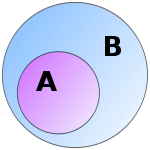• "Red and black at the same time without being a joker" (0 elements),
• "The 5 of Hearts" (1 element),
• "A King" (4 elements),
• "A Face card" (12 elements),
• "A Face card or a red suit" (32 elements),
• "A card" (52 elements).

Since all events are sets, they are usually written as sets (e.g. {1, 2, 3}), and represented graphically using Venn diagram
Venn diagram
Venn diagrams or set diagrams are diagrams that show all possible logical relations between a finite collection of sets . Venn diagrams were conceived around 1880 by John Venn...

s. Given that each outcome in the sample space Ω is equally likely, the probability of an event A is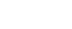this rule can readily be applied to each of the example events above.

## Events in probability spaces

Defining all subsets of the sample space as events works well when there are only finitely many outcomes, but gives rise to problems when the sample space is infinite. For many standard probability distributions, such as the normal distribution, the sample space is the set of real numbers or some subset of the real numbers. Attempts to define probabilities for all subsets of the real numbers run into difficulties when one considers 'badly-behaved'
Pathological (mathematics)
In mathematics, a pathological phenomenon is one whose properties are considered atypically bad or counterintuitive; the opposite is well-behaved....

sets, such as those that are nonmeasurable. Hence, it is necessary to restrict attention to a more limited family of subsets. For the standard tools of probability theory, such as joint and conditional probabilities
Conditional probability
In probability theory, the "conditional probability of A given B" is the probability of A if B is known to occur. It is commonly notated P, and sometimes P_B. P can be visualised as the probability of event A when the sample space is restricted to event B...

, to work, it is necessary to use a σ-algebra
Sigma-algebra
In mathematics, a σ-algebra is a technical concept for a collection of sets satisfying certain properties. The main use of σ-algebras is in the definition of measures; specifically, the collection of sets over which a measure is defined is a σ-algebra...

, that is, a family closed under complementation and countable unions of its members. The most natural choice is the Borel measurable set derived from unions and intersections of intervals. However, the larger class of Lebesgue measurable
Lebesgue measure
In measure theory, the Lebesgue measure, named after French mathematician Henri Lebesgue, is the standard way of assigning a measure to subsets of n-dimensional Euclidean space. For n = 1, 2, or 3, it coincides with the standard measure of length, area, or volume. In general, it is also called...

sets proves more useful in practice.

In the general measure-theoretic description of probability space
Probability space
In probability theory, a probability space or a probability triple is a mathematical construct that models a real-world process consisting of states that occur randomly. A probability space is constructed with a specific kind of situation or experiment in mind...

s, an event may be defined as an element of a selected σ-algebra
Sigma-algebra
In mathematics, a σ-algebra is a technical concept for a collection of sets satisfying certain properties. The main use of σ-algebras is in the definition of measures; specifically, the collection of sets over which a measure is defined is a σ-algebra...

of subsets of the sample space. Under this definition, any subset of the sample space that is not an element of the σ-algebra is not an event, and does not have a probability. With a reasonable specification of the probability space, however, all events of interest are elements of the σ-algebra.

## A note on notation

Even though events are subsets of some sample space Ω, they are often written as propositional formula
Propositional formula
In propositional logic, a propositional formula is a type of syntactic formula which is well formed and has a truth value. If the values of all variables in a propositional formula are given, it determines a unique truth value...

s involving random variable
Random variable
In probability and statistics, a random variable or stochastic variable is, roughly speaking, a variable whose value results from a measurement on some type of random process. Formally, it is a function from a probability space, typically to the real numbers, which is measurable functionmeasurable...

s. For example, if X is a real-valued random variable defined on the sample space Ω, the event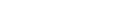can be written more conveniently as, simply,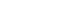This is especially common in formulas for a probability
Probability
Probability is ordinarily used to describe an attitude of mind towards some proposition of whose truth we arenot certain. The proposition of interest is usually of the form "Will a specific event occur?" The attitude of mind is of the form "How certain are we that the event will occur?" The...

, such as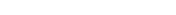The set u < Xv is an example of an inverse image under the mapping
Map (mathematics)
In most of mathematics and in some related technical fields, the term mapping, usually shortened to map, is either a synonym for function, or denotes a particular kind of function which is important in that branch, or denotes something conceptually similar to a function.In graph theory, a map is a...

X because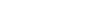if and only if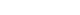.